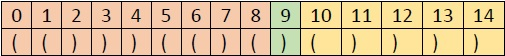# Find an equal point in a string of brackets using C++.

C++Server Side ProgrammingProgramming

Here we will see how to get the equal points in a string of brackets. The equal point is the index I, such that the number of opening brackets before it is equal to the number of the closing bracket after it. Suppose a bracket string is like “(()))(()()())))”, if we see closer, we can getSo the number of opening brackets from 0 to 9 is 5, and the number of the closing brackets from 9 to 14 is also 5, so this is the equal point.

To solve this problem, we have to follow these few steps −

• Store the number of opening brackets appears in the string up to every index i
• Store the number of closing brackets appears in the string up to each and every index I but it should be done from the last index.
• Check whether an index has the same value of opening and closing brackets.

## Example

#include<iostream>
#include<cmath>
using namespace std;
int findEqualPoint(string str) {
int total_length = str.length();
int open[total_length+1] = {0}, close[total_length+1] = {0};
int index = -1;
open = 0;
close[total_length] = 0;
if (str=='(') //check whether first bracket is opening or not, if so mark open = 1
open = 1;
if (str[total_length-1] == ')') //check whether first bracket is closing or not, if so mark       close[end] = 1
close[total_length-1] = 1;
for (int i = 1; i < total_length; i++) {
if ( str[i] == '(' )
open[i+1] = open[i] + 1;
else
open[i+1] = open[i];
}
for (int i = total_length-2; i >= 0; i--) {
if ( str[i] == ')' )
close[i] = close[i+1] + 1;
else
close[i] = close[i+1];
}
if (open[total_length] == 0)
if (close == 0)
return 0;
for (int i=0; i<=total_length; i++)
if (open[i] == close[i])
index = i;
return index;
}
int main() {
string str = "(()))(()()())))";
cout << "Index of equal point: " << findEqualPoint(str);
}

## Output

Index of equal point: 9